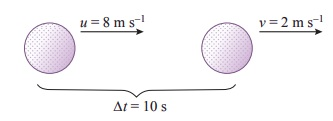Home | | Science 7th Std | Acceleration

# Acceleration

Acceleration is the rate of change in velocity. In other words if a body changes its speed or dirction then it is said to be accelerated.

Acceleration

Acceleration (a)

Acceleration is the rate of change in velocity. In other words if a body changes its speed or dirction then it is said to be accelerated.

Acceleration = change in velocity/ time = [final velocity (v) â€“ intial velocity (u) ] / time (t)

a = (vâ€“u) / t

SI unit of acceleration is m/s2

In other words, the object undergoes acceleration when its speed and/or direction change(s).The velocity at different times of a train departing direction is given in the figure. Analyse this and complete the table .Analysis:

When the train covers the distance A to B and B to C, it is accelerated motion.

When it covers the distance C-D, there is no acceleration (i.e) uniform velocity.

When it covers the distance D to E and E to F it has negative acceleration or deceleration or retardation, (i.e.) Its velocity decreases with respect to time.

Tell meA car at rest starts to travel in a straight path. It reaches a velocity of 12 m/s in 4 s . What is its acceleration. Assuming that it accelerates uniformly?

Initial velocity u      =0 m/s (since the car starts from rest)

Final velocity (v)      =12 m /s

Time taken (t )           =4 s

acceleration ( a )       =(v â€“ u) / t

= (12â€“0)/4

=3 m / s2

See how brisk I am !My name is cheetah. I can run at a great speed. Do you know what my speed is? 25 m/s to 30 m/s. My speed changes from 0 to 20 m/s in 2 second. See how good my acceleration is !

Acceleration of the cheetah = [ Final velocity(v) - Initial velocity(u) ] / [time taken]

= [ v - u ] / t = 20-0 / 2 = 20/2 = 10 m/s2

Acceleration of the cheetah = 10 m/s2

Tell me

From the above information, can you calculate the acceleration of the cheetah?

Positive acceleration

If the velocity of an object increases with respect to time, then the object is said to be in positive acceleration or just acceleration.

Negative acceleration or deceleration or retardation

If the velocity of an object decreases with respect to time, then the object is said to be in negative acceleration or deceleration or retardation.The velocity of a golf ball rolling in a straight line changes from 8 m/s to 2 m/s in 10 s. What is its deceleration, assuming that it is decelerating uniformly ?

Initial velocity (u)      =8 m/s

Final velocity (v)       =2 m/s

Time taken(t)             =10 s

Acceleration (a)          =(v â€“ u)/t

=(2 â€“ 8)/10

= â€“0.6 m/s2

The deceleration is â€“0.6 m/s2

Uniform acceleration

An object undergoes uniform acceleration when the change (increase or decrease) in its velocity for every unit of time is the same.

Table shows a moving bus with uniform acceleration.When the velocity of the object is increasing by 20 m/s the acceleration is 20 m/s2  When the velocity of the object is decreasing by 20 m/s the deceleration is 20 m/s2.

When the velocity of the object is decreasing by 20m/s the deceleration is 20 m/s2.

Deceleration = Decrease in velocity / Deceleration = t = 20/20 = 1s

The velocity of the object is decreasing by 20m/s in one second.

Non â€“ uniform acceleration

An object undergoes non uniform acceleration if the change in its velocity for every unit of time is not the same.Note that the change in velocity is not the same for every second. The moving object is undergoing non uniform acceleration.

Tags : Force and Motion | Term 1 Unit 2 | 7th Science , 7th Science : Term 1 Unit 2 : Force and Motion
Study Material, Lecturing Notes, Assignment, Reference, Wiki description explanation, brief detail
7th Science : Term 1 Unit 2 : Force and Motion : Acceleration | Force and Motion | Term 1 Unit 2 | 7th Science